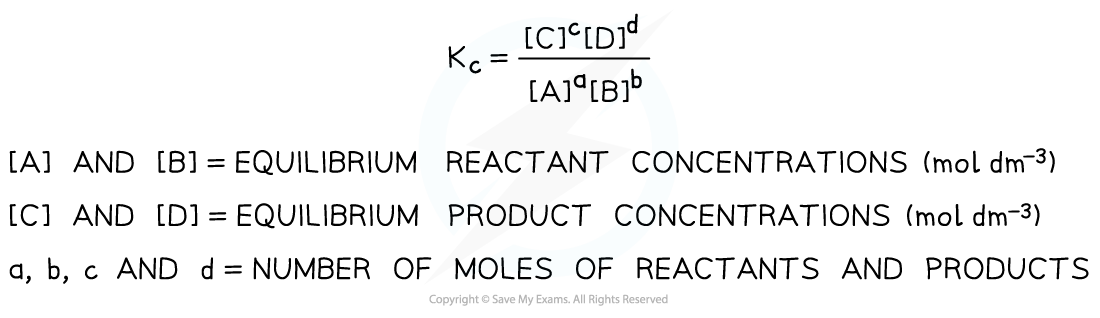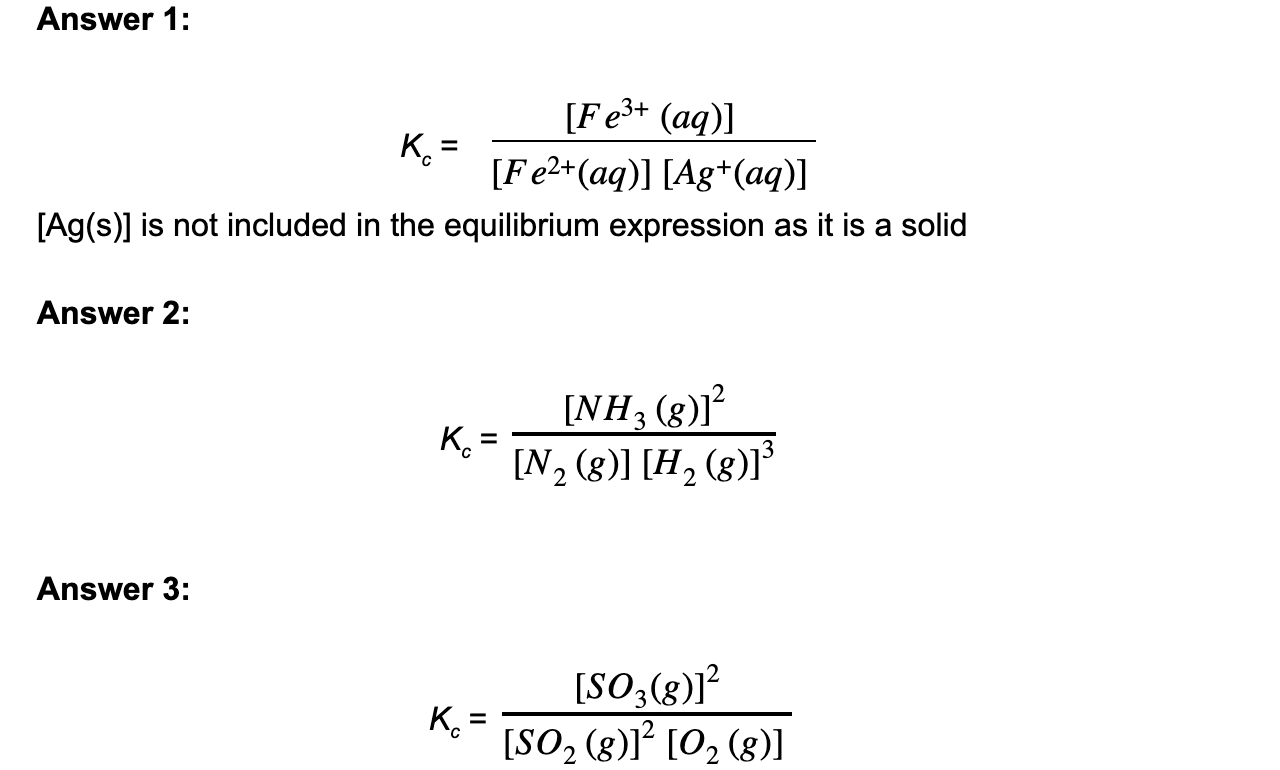# IB DP Chemistry: SL复习笔记7.1.2 The Equilibrium Law

### The Equilibrium Constant

#### Equilibrium expression & constant

• The equilibrium expression is an expression that links the equilibrium constant, Kc, to the concentrations of reactants and products at equilibrium taking the stoichiometry of the equation into account
• So, for a given reaction:

aA + bB ⇌ cC + dD

the Kc is expressed as follows:Equilibrium expression linking the equilibrium concentration of reactants and products at equilibrium

• Solids are ignored in equilibrium expressions
• The Kc of a reaction is specific to a given equation

#### Worked Example

Deduce the equilibrium constant expression for the following reactions

1. Ag+ (aq) + Fe2+ (aq) ⇌ Ag (s) + Fe3+ (aq)
2. N2 (g) + 3H2 (g) ⇌ 2NH3 (g)
3. 2SO2 (g) + O2 (g) ⇌ 2SO3 (g)### The Size of Kc

• The size of Ktells us how the equilibrium mixture is made up with respect to reactants and products• If Kc > 1, the concentration of products is greater than the concentration of reactants and we say that the equilibrium lies to the right hand side
• If Kc < 1, then the concentration of reactants is greater than the concentration of products and we say that the equilibrium lies to the left hand side
• Kcis a constant at a specified temperature
• Since temperature can affect the position of equilibrium it follows that Kc is dependent on temperature

#### Worked Example

When the following reactions reach equilibrium, state whether the equilibrium mixture contains mostly reactants or products.Assume the value of Kcorresponds to the temperature of the reaction mixture

1. Ag+ (aq) + Fe2+ (aq) ⇌ Ag (s) + Fe3+ (aq)    Kc = 7.3 x 10-26
2. N2 (g) + 3H2 (g) ⇌ 2NH3 (g)                           Kc = 2.6 x 10-18
3. 2SO2 (g) + O2 (g) ⇌ 2SO3 (g)                         Kc = 5.0 x 1013

• 1 and 2: As Kis very much smaller than 1 the denominator in the equilibrium expression must be much larger than the numerator so the concentration of the reactants is much larger than the concentration of products. The equilibrium mixture contains mostly reactants
• 3: As Kis very much larger than 1 the numerator in the equilibrium expression must be much larger than the denominator so the concentration of the products is much larger than the concentration of reactants. The equilibrium mixture contains mostly products